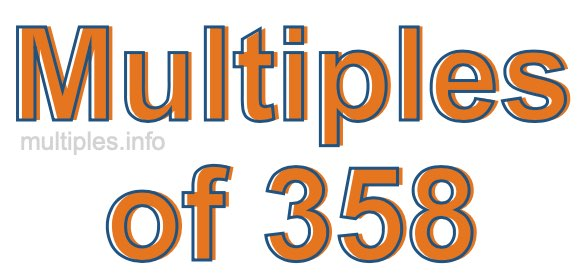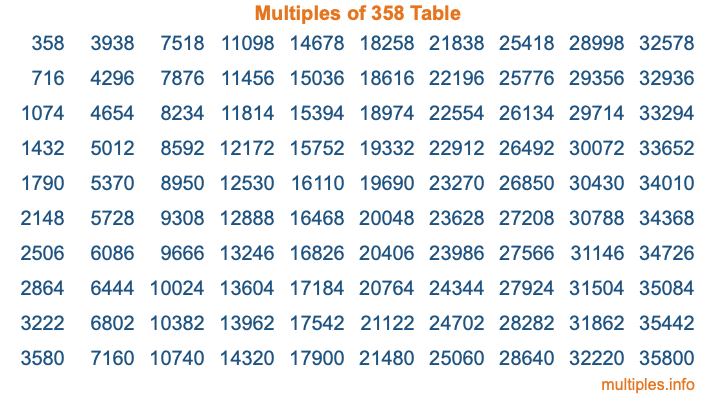Multiples of 358Welcome to the Multiples of 358 page. Here we will first teach you everything you will ever need to know about the multiples of 358, and then give you a study guide summary of everything we taught you to make sure you remember it all. Use this page to look up facts and learn information about the multiples of 358. This page will make you a multiples of three hundred fifty-eight expert!

Definition of Multiples of 358
Multiples of 358 are all the numbers that when divided by 358 equal an integer. Each of the multiples of 358 are called a multiple. A multiple of 358 is created by multiplying 358 by an integer.

Therefore, to create a list of multiples of 358, you start with 1 multiplied by 358, then 2 multiplied by 358, then 3 multiplied by 358, and so on for as long as you want. Thus, the list of the first five multiples of 358 is 358, 716, 1074, 1432, and 1790. To see a larger list of multiples of 358, see the printable image of Multiples of 358 further down on this page. We also have a category where you can choose any nth multiple of 358.

Multiples of 358 Checker
The Multiples of 358 Checker below checks to see if any number of your choice is a multiple of 358. In other words, it checks to see if there is any number (integer) that when multiplied by 358 will equal your number. To do that, we divide your number by 358. If the the quotient is an integer, then your number is a multiple of 358.

Is  a multiple of 358?

Least Common Multiple of 358 and ...
A Least Common Multiple (LCM) is the lowest multiple that two or more numbers have in common. This is also called the smallest common multiple or lowest common multiple and is useful to know when you are adding our subtracting fractions. Enter one or more numbers below (358 is already entered) to find the LCM.

Check out our LCM Calculator if you need more details about the Least Common Multiple or if you need the LCM for different numbers for adding and subtraction fractions.

nth Multiple of 358
As we stated above, 358 is the first multiple of 358, 716 is the second multiple of 358, 1074 is the third multiple of 358, and so on. Enter a number below to find the nth multiple of 358.

th multiple of 358

Multiples of 358 vs Factors of 358
358 is a multiple of 358 and a factor of 358, but that is where the similarities end. All postive multiples of 358 are 358 or greater than 358. All positive factors of 358 are 358 or less than 358.

Below is the beginning list of multiples of 358 and the factors of 358 so you can compare:

Multiples of 358: 358, 716, 1074, 1432, 1790, etc.

Factors of 358: 1, 2, 179, 358

As you can see, the multiples of 358 are all the numbers that you can divide by 358 to get a whole number. The factors of 358, on the other hand, are all the whole numbers that you can multiply by another whole number to get 358.

It's also interesting to note that if a number (x) is a factor of 358, then 358 will also be a multiple of that number (x).

Multiples of 358 vs Divisors of 358
The divisors of 358 are all the integers that 358 can be divided by evenly. Below is a list of the divisors of 358.

Divisors of 358: 1, 2, 179, 358

The interesting thing to note here is that if you take any multiple of 358 and divide it by a divisor of 358, you will see that the quotient is an integer.

Multiples of 358 Table
Below is an image of the first 100 multiples of 358 in a table. The table is in chronological order, column by column. The first column has the first ten multiples of 358, the second column has the next ten multiples of 358, and so on.The Multiples of 358 Table is also referred to as the 358 Times Table or Times Table of 358. You are welcome to print out our table for your studies.

Negative Multiples of 358
Although not often discussed or needed in math, it is worth mentioning that you can make a list of negative multiples of 358 by multiplying 358 by -1, then by -2, then by -3, and so on, to get the following list of negative multiples of 358:

-358, -716, -1074, -1432, -1790, etc.

Multiples of 358 Summary
Below is a summary of important Multiples of 358 facts that we have discussed on this page. To retain the knowledge on this page, we recommend that you read through the summary and explain to yourself or a study partner why they hold true.

There are an infinite number of multiples of 358.

A multiple of 358 divided by 358 will equal a whole number.

358 divided by a factor of 358 equals a divisor of 358.

The nth multiple of 358 is n times 358.

The largest factor of 358 is equal to the first positive multiple of 358.

358 is a multiple of every factor of 358.

358 is a multiple of 358.

A multiple of 358 divided by a divisor of 358 equals an integer.

358 divided by a divisor of 358 equals a factor of 358.

Any integer times 358 will equal a multiple of 358.

Multiples of a Number
Here you can get the multiples of another number, all with the same attention to detail as we did for multiples of 358 on this page.

Multiples of
Multiples of 359
Did you find our page about multiples of three hundred fifty-eight educational? Do you want more knowledge? Check out the multiples of the next number on our list!